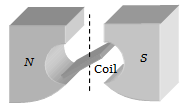# NEET Physics Magnetism and Matter Questions Solved

The figure below shows the north and south poles of a permanent magnet in which n turn coil of area of cross-section A is resting, such that for a current i passed through the coil, the plane of the coil makes an angle with respect to the direction of magnetic field B. If the plane of the magnetic field and the coil are horizontal and vertical respectively, the torque on the coil will be(a)τ = $niAB\mathrm{cos}\theta$

(b)τ = $niAB\mathrm{sin}\theta$

(c)τ = niAB

(d) None of the above, since the magnetic field is radial